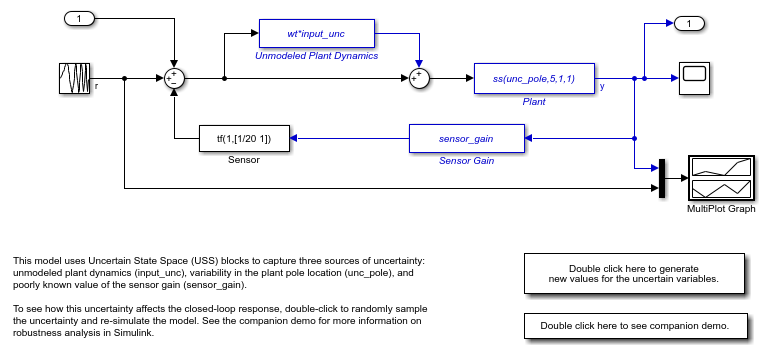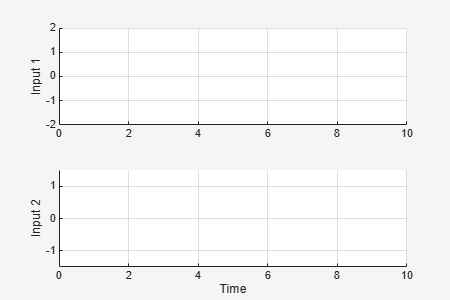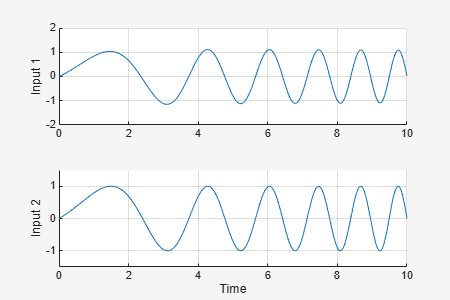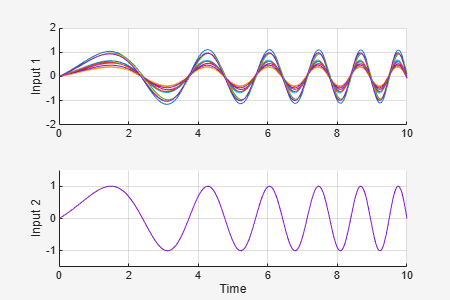# usample

Generate random samples of uncertain variables in a Simulink model

## Syntax

``uvals = usample(uvars)``
``uvals = usample(uvars,N)``
``uvals = usample(uvars,N,wmax)``

## Description

Use this function to generate random samples of uncertain variables in a Simulink® model that contains Uncertain State Space blocks. Such sampling lets you perform Monte Carlo analysis of the model response.

To generate random samples from uncertain elements and models such as `uss`, `ufrd`, `genss`, or `genfrd` models, use `usample`.

example

````uvals = usample(uvars)` generates a random sample of the uncertain variables in the structure `uvars`. This structure lists the uncertain variables in a Simulink model that contains Uncertain State Space blocks. To obtain the input `uvars`, for a particular Simulink model, use `ufind`. The resulting structure `uvals` has fields whose names are the uncertain variable names and values are the corresponding samples. To apply the sampled values when you simulate the model, set the Uncertainty value parameter of the Uncertain State Space block to `uvars`.```
````uvals = usample(uvars,N)` takes `N` samples and returns them in an `N`-by-1 structure array. ```
````uvals = usample(uvars,N,wmax)` restricts the maximum frequency of poles in the sample when `uvars` contains uncertain dynamic elements `ultidyn` or `umargin`. For details, see the `wmax` argument description.```

## Examples

collapse all

Generate random samples of uncertain variables in a Simulink model.

Open the model.

```open_system('usim_model') ```The model contains three Uncertain State Space blocks named `Unmodeled Plant Dynamics`, `Plant`, and `Sensor Gain`. Each of these blocks is preconfigured with an uncertain system variable that depends on an uncertain element, as follows.

• Unmodeled plant dynamics: a `ultidyn` element named `input_unc`

• Plant: a `ureal` element called `unc_pole`

• Sensor gain: a `ureal` element called `sensor_gain`

Create a structure of these uncertain elements using `ufind`. This command finds all Uncertain State Space blocks and uncertain variables in the model.

```uvars = ufind('usim_model'); uvars ```
```uvars = struct with fields: input_unc: [1x1 ultidyn] sensor_gain: [1x1 ureal] unc_pole: [1x1 ureal] ```The field names and values of `uvars` correspond to the uncertain elements in the three Uncertain State Space blocks. To simulate the model at random values of these variables, use `usample` to generate the values in a structure.

```uval = usample(uvars) ```
```uval = struct with fields: input_unc: [1x1 ss] sensor_gain: 0.2853 unc_pole: -8.3290 ```

Each field of `uval` contains the corresponding sample value. The randomly chosen values of the `ureal` elements are real scalar values. The random sample of the `ultidyn` element is a state-space model.

To simulate the model using these random values, set the Uncertainty value parameter of each Uncertain State Space block to `uval`. In `usim_model` this parameter is already set. Therefore, when you simulate the model, Simulink applies the values in `uval` to the uncertain variables in the model.

```sim('usim_model') ```For Monte Carlo analysis, you can use a `for` loop to repeatedly sample the variables and simulate the model.

```for i=1:10; uval = usample(uvars); sim('usim_model',10); end ```The MultiPlot Graph block displays the simulated responses.

## Input Arguments

collapse all

Uncertain variables to sample, specified as a structure whose field names are the names of uncertain variables and whose field values are the corresponding uncertain elements. For instance, `uvars` might have a field `a` whose value is a `ureal` element and a field `Delta` whose value is a `ultidyn` element.

To sample uncertain variables in a Simulink model that contains Uncertain State Space blocks, use the `ufind` command to construct `uvars`. This command finds the uncertain variables referenced in the Uncertain State Space blocks in the model and populates the names and values of the structure `uvars` accordingly.

Number of samples to take, specified as a positive integer.

Maximum magnitude of poles in sampled dynamics, specified as a positive scalar value. When you sample uncertain dynamics (`ultidyn`) or gain and phase uncertainty (`umargin`), use this input to limit the frequency of poles in the resulting system.

For `ultidyn` variables with `Type` set to `GainBounded` and `umargin` variables:

• If variable is continuous time (`Ts = 0`), then each pole of the sampled dynamics has a natural frequency less than or equal to `wmax`.

• If the variable is discrete time (`Ts != 0`), then the natural frequency of each pole is less than `min(wmax,1/(2*Ts))`.

For `ultidyn` variables with `Type` set to `PositiveReal`, `usample` samples the variables as in the `GainBounded` case and performs a bilinear transform on the result.

The value of `wmax` overrides the `SampleMaxFrequency` property of the `umargin` or `ultidyn` variable.

To control the order of the sampled dynamics, use the `SampleStateDimension` property of the`umargin` or `ultidyn` variable.

## Output Arguments

collapse all

Sampled values of uncertain elements, returned as an `N`-by-1 structure array whose field names are the same as those in `uvars` and whose values are the randomly-chosen specific (not-uncertain) values of the corresponding uncertain elements.

## Version History

Introduced in R2009b